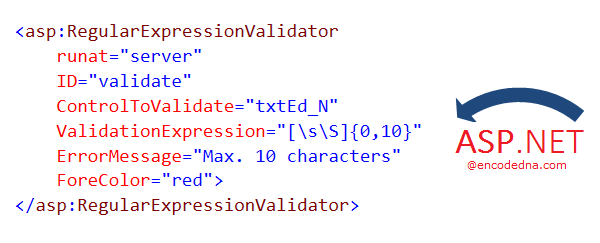﻿ How to use Asp.Net RegularExpressionValidator on a Textbox

# How to use Asp.Net RegularExpressionValidator on a Textbox

There are many ways you can validate a textbox at the client side itself. However, it is advisable to validate the contents of the textbox at the server side also, before saving it. If you are using an Asp.Net application, you can use the RegularExpressionValidator control to validate the value in a textbox control.Using RegularExpressionValidator control, you can validate or check several types of input values, like, validating numbers only, or check if it’s a valid e-mail id etc.

I am sharing few examples here that show how you can easily validate values in an Asp.Net textbox control with the RegularExpressionValidator control.

## Example 1: Validate a 10 digit alphanumeric value in a Textbox

In the first example, I have a textbox control and I want my users to enter only a 10 digit alphanumeric value in it. If it exceeds the limit, it would show a message.

```<p>
<asp:TextBox ID="txtN"
placeholder="Enter some text here [Max. 10 Chars.]"
Width="300px"
runat="server">
</asp:TextBox>
</p>

<asp:RegularExpressionValidator
runat="server"
ID="validate"
ControlToValidate="txtN"
ValidationExpression="[\s\S]{0,10}"
ErrorMessage="Please enter a maximum of 10 characters"
ForeColor="red">
</asp:RegularExpressionValidator>```

Pay attention to the ValidationExpression property of the RegularExpressionValidator control. The first two MetaCharacters checks for white-space characters (that is \s) and non white-space characters (that is \S) and these are inside a square bracket.

Next, inside the curly braces, I have defined the character limit, between 0 and 10. So, if a user enters some value in the textbox that exceeds the 10 digit character limit, validator control will show a message in red.

ErrorMessage="Please enter a maximum of 10 characters"
ForeColor="red"

Until it is satisfied that the entered value is in the prescribed limit, you cannot save the values or it will not send the input values to the server. To check this, simply add a button control and write a small code.

```<p>
<asp:TextBox ID="txtN"
placeholder="Enter some text here [Max. 10 Chars.]"
Width="300px"
runat="server">
</asp:TextBox>
</p>

<%--Regular Expression Validator control--%>
<asp:RegularExpressionValidator
runat="server"
ID="validate"
ControlToValidate="txtN"
ValidationExpression="[\s\S]{0,10}"
ErrorMessage="Please enter a maximum of 10 characters"
ForeColor="red">
</asp:RegularExpressionValidator>

<%--Button control--%>
<p>
<asp:Button ID="Button1" Text="Save Data" OnClick="validateText" runat="server" />
</p>```
Code behind C#
```protected void validateText(object sender, EventArgs e)
{
}```
Vb.Net
```Protected Sub validateText(sender As Object, e As EventArgs)
MsgBox(txtN.Text)
End Sub```

## Example 2: Validate a 10 digit Number only value in Textbox

In the previous example, the validator checked for alphanumeric values in the textbox. Here, we’ll validate the textbox for numbers only that too with a limit, lets say, a 10 digit number.

For a 10-digit number only test, we can use any cell phone number.

```<p>
<asp:TextBox ID="txtMobile"
placeholder="Enter mobile number"
Width="300px"
runat="server">
</asp:TextBox>
</p>

<asp:RegularExpressionValidator
runat="server"
ID="validate"
ControlToValidate="txtMobile"
ValidationExpression="\d{10}"
ErrorMessage="Enter a 10 digit mobile number"
ForeColor="red">
</asp:RegularExpressionValidator>```
The validator will keep showing the error message, until the user enters a valid 10 digit number only value. The value cannot be less than 10. The message will not appear when its satisfied with value or the pattern.

## Example 3: Validate any 5 digit Number only value between 0 to 9

Here’s another example to validate textbox values. We’ll set a limit a 5 digit number only value and the numbers can only be between 0 to 9

```<p>
<asp:TextBox ID="txtN"
placeholder="Enter a 5 digit number between 0 and 9"
Width="300px"
runat="server">
</asp:TextBox>
</p>

<asp:RegularExpressionValidator
runat="server"
ID="validate"
ControlToValidate="txtN"
ValidationExpression="^[0-9]{5}\$"
ErrorMessage="Invalid!"
ForeColor="red">
</asp:RegularExpressionValidator>```
Conclusion

The 3 examples that I have shared shows how using the RegularExpressionValidator control, you can easily validate the values inside a textbox control at the client side. The procedure is simple. However, you'll have to learn to use the expressions (like \d{10}) to do different validation. The examples here also shows some commonly used RegEx (regular expressions) that you can use with the validator to check the data in an input control.